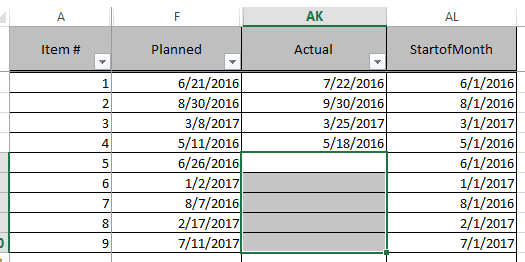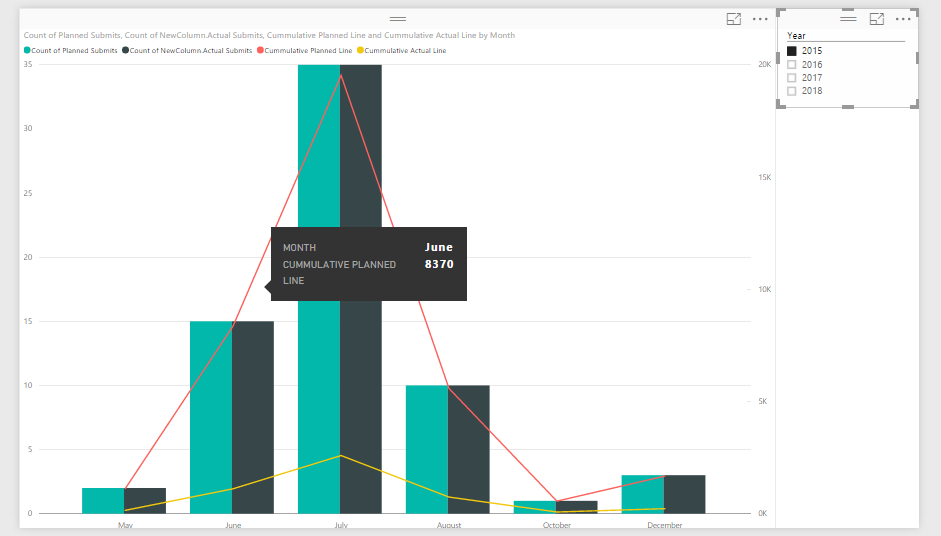cancel
Showing results for
Did you mean:Frequent Visitor

## Cumulative Line Formula

Hello. I need to draw a cumulative line across this graph for both columns represented as bars in this graph visual:Both columns are date columns and are on the same table, and the bars represent the count of the dates entered in the table in each month. The formula for this line is the same for both columns, and in plain text is pretty simple (Count of January dates, Count of January dates + Count of February dates, Count of January dates + Count of February dates + Count of March dates, and so on…).

I'm having trouble writing a DAX formula that would produce the desired result. I've tried quite a few of the formulas I've seen listed on this forum but have not had luck.

1 ACCEPTED SOLUTIONYou should be able to use the formula that @Greg_Deckler provided and just change the SUM to a COUNT of [Planned] or [Actual].

```Cummulative Actual Line =
CALCULATE (
COUNTA ( 'Table1'[Actual] ),
FILTER (
ALL ( 'Table1' ),
'Table1'[StartofMonth] <= MAX ( 'Table1'[StartofMonth] )
)
)Cummulative Planned Line =CALCULATE (    COUNTA ( 'Table1'[Planned] ),    FILTER (        ALL ( 'Table1' ),        'Table1'[StartofMonth] <= MAX ( 'Table1'[StartofMonth] )    ))```

Here is what I generated with your example data set.11 REPLIES 11Regular Visitor

This keeps coming up first in Google for me, so I thought I'd leave this here for future users. the Power BI team have released the Quick Measures Preview, which among other things includes a quick calculation for running totals (as well as various Time Intelligence formulas like Year to Date)Helper I

Hi!

I have this DAX formula and it doesn't works for me, what am I doing wrong?

Thanks so much!!

SUM ( 'InvoiceSet'[Amount PreTax] );
FILTER (
ALL ( InvoiceSet[Invoice Date] );
InvoiceSet[Invoice Date] <= MAX ( InvoiceSet[Invoice Date] )
)
)Community Champion

EL filtro debe de ir la tabla

FILTER (
ALL ( InvoiceSet)

Lima - PeruSuper User

The general cumulative total pattern can be found here:

http://www.daxpatterns.com/cumulative-total/

Basically it is:

```Cumulative Quantity :=
CALCULATE (
SUM ( Transactions[Quantity] ),
FILTER (
ALL ( 'Date'[Date] ),
'Date'[Date] <= MAX ( 'Date'[Date] )
)
)```

I would have to see your specific data, or sample data and relationships to write a specific solution or your model.

Become an expert!: Enterprise DNA
External Tools: MSHGQM
Latest book!:
Mastering Power BI 2nd EditionDAX is easy, CALCULATE makes DAX hard...Anonymous
Not applicable

I used the formula but it is not limiting based on the Visual Filter.

Here is my formula:

Rev_Local_Cummulative =
CALCULATE (

_Revenue[Revenue_LocalCurrency] ,

FILTER (
ALL ( DateInvoice[CalendarDate] ),
'DateInvoice'[CalendarDate] <= MAX ( 'DateInvoice'[CalendarDate] )
)
)

I have a Visualization Filter set to June 2018. June 1 starts out with the sum of all prior data and then increments by the current filtered month daily amount.New Member

Thanks, this worked perfectly for what I needed.Frequent Visitor

Here a sample of the data&colon;The report I showed an image of has bars that count the number of dates in the "Planned" and "Actual" columns and show them in bars to show the difference in the two counts. The "StartOfMonth" column is used to group the bars together by each month in the 'Shared Axis' field of the BI report. All columns are in one table.You should be able to use the formula that @Greg_Deckler provided and just change the SUM to a COUNT of [Planned] or [Actual].

```Cummulative Actual Line =
CALCULATE (
COUNTA ( 'Table1'[Actual] ),
FILTER (
ALL ( 'Table1' ),
'Table1'[StartofMonth] <= MAX ( 'Table1'[StartofMonth] )
)
)Cummulative Planned Line =CALCULATE (    COUNTA ( 'Table1'[Planned] ),    FILTER (        ALL ( 'Table1' ),        'Table1'[StartofMonth] <= MAX ( 'Table1'[StartofMonth] )    ))```

Here is what I generated with your example data set.Frequent Visitor

I implemented the formulas suggested above, but the numbers illustrated don't seem to match up to the numbers on the bars.You can see the line value at the highlighted point is 8,370 while the bars is hovers by are both only showing a value of 15. It seems to just be doubling it's count of every item in the data set (there are about 550 total items in the data set by their dates span over a long period of time).

Did I miss a step?

Judging by the image @Twan showed of his solution, that formula should work perfectly.Microsoft

what is the exact formula that you used... can you provide that?Frequent Visitor

@Twan's formula was the solution. Accidentally applied the formula as a column, but it worked fine when I applied it to a measure.

Thanks to everyone who helped!